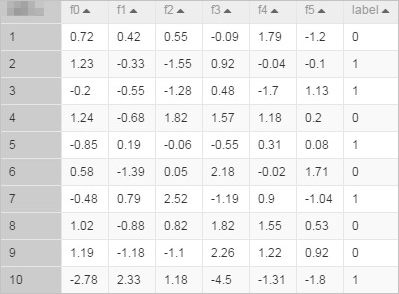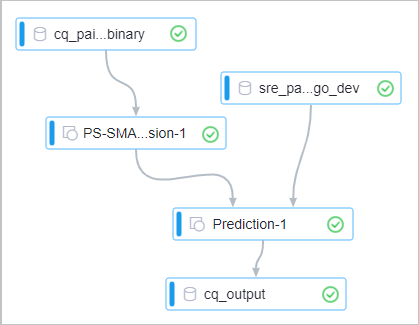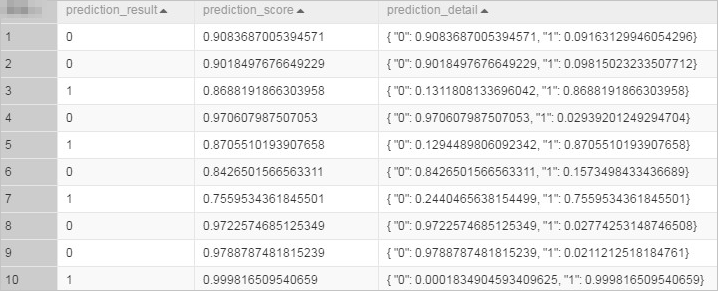A parameter server (PS) is used to process a large number of offline and online training tasks. Logistic Regression is a classical binary classification algorithm. It is widely used in advertising and searching scenarios. PS Logistic Regression supports training tasks of binary classification for hundreds of billions of samples and billions of features.

## Limits

The input data of the PS Logistic Regression for Binary Classification component must meet the following requirements:
• Only columns of numeric data types can be used by the PS Logistic Regression for Binary Classification component. 0 indicates a negative example, and 1 indicates a positive example. If the type of data in the MaxCompute table is STRING, the data type must be converted first. For example, you must convert Good/Bad to 1/0.
• If data is in the key-value format, feature IDs must be positive integers, and feature values must be real numbers. If the data type of feature IDs is STRING, you must use the serialization component to serialize the data. If feature values are categorical strings, you must perform feature engineering such as feature discretization to process the values.

## Configure the component

You can configure the component by using one of the following methods:
• Use the Machine Learning Platform for AI console
Tab Parameter Description
Fields Setting Feature Columns The feature columns that are selected from the data source for training. If data in the input table is in the dense format, columns of the DOUBLE and BIGINT types are supported. If data in the input table is key-value pairs in the sparse format, only columns of the STRING type are supported.
Label Column The name of the label column in the input table.
Use Sparse Format Specifies whether the input data is in the sparse format. If the Use Sparse Format parameter is set to Yes, the feature ID cannot be 0. We recommend that you number features from 1.
Parameters Setting L1 weight The L1 regularization coefficient. The larger the value of this parameter is, the fewer non-zero elements the model has. If overfitting occurs, increase the parameter value.
L2 weight The L2 regularization coefficient. The larger the value of this parameter is, the smaller the absolute values of the model parameters are. If overfitting occurs, increase the parameter value.
Maximum Iterations The maximum number of iterations of the algorithm. If this parameter is set to 0, the number of iterations is unlimited.
Minimum Convergence Deviance The conditions to terminate the algorithm. The value of this parameter is the average value of the relative change rates of losses for 10 iterations. The smaller the value of this parameter is, the longer the algorithm runs.
Largest Feature ID The maximum feature ID or feature dimension. The value of this parameter can be greater than the actual value. The larger the value of this parameter is, the more the memory size will be used. If this parameter is not specified, the system automatically runs an SQL task to calculate the maximum feature ID or feature dimension.
Tuning Cores The number of cores.
Memory Size per Core The memory size of each core. Unit: MB.
• Use commands
``````# Training
PAI -name ps_lr
-project algo_public
-DinputTableName="lm_test_input"
-DmodelName="logistic_regression_model"
-DlabelColName="label"
-DfeatureColNames="f0,f1,f2,f3,f4,f5"
-Dl1Weight=1.0
-Dl2Weight=0.0
-DmaxIter=100
-Depsilon=1e-6
-DenableSparse=false
# Prediction
drop table if exists logistic_regression_predict;
PAI -name prediction
-DmodelName="logistic_regression_model"
-DoutputTableName="logistic_regression_predict"
-DinputTableName="lm_test_input"
-DappendColNames=label
-DfeatureColNames="f0,f1,f2,f3,f4,f5"
-DenableSparse=false``````
Parameter Required Description Default value
inputTableName Yes The name of the input table. N/A
featureColNames Yes The feature columns that are selected from the input table for training. If data in the input table is in the dense format, columns of the DOUBLE and BIGINT types are supported. If data in the input table is key-value pairs in the sparse format, only columns of the STRING type are supported. N/A
labelColName Yes The label column that is selected from the input table. The columns of the DOUBLE and BIGINT types are supported. N/A
inputTablePartitions No The partitions that are selected from the input table for training. Specify this parameter in one of the following formats:
• partition_name=value
• name1=value1/name2=value2: multi-level partitions
Note If you specify multiple partitions, separate them with commas (,).
Full table
modelName Yes The name of the output model. By default, the model is generated as an offline model. If the enableModelIo parameter is set to false, the model is generated in MaxCompute. N/A
enableModelIo No Specifies whether the model is generated as an offline model. Valid values: true and false. If the enableModelIo parameter is set to false, the model is generated in MaxCompute. true
l1Weight No The L1 regularization coefficient. The larger the value of this parameter is, the fewer non-zero elements the model has. If overfitting occurs, increase the parameter value. 1.0
l2Weight No The L2 regularization coefficient. The larger the value of this parameter is, the smaller the absolute values of the model parameters are. If overfitting occurs, increase the parameter value. 0
maxIter No The maximum number of iterations of the algorithm. 100
epsilon No The conditions to terminate the algorithm. 1.0e-06
modelSize No The maximum feature ID or feature dimension. The value of this parameter can be greater than the actual value. The larger the value of this parameter is, the more the memory size will be used. If this parameter is not specified, the system automatically runs an SQL task to calculate the maximum feature ID or feature dimension. 0
enableSparse No Specifies whether data in the input table is in the sparse format. Valid values: true and false. false
itemDelimiter No The delimiter that is used to separate key-value pairs if data in an input table is in the sparse format. ,
kvDelimiter No The delimiter that is used to separate keys and values if data in an input table is in the sparse format. :
coreNum No The number of cores. Determined by the system
memSizePerCore No The memory size of each core. Unit: MB. Determined by the system

## Example

1. Execute the following SQL statements to generate training data. In this example, training data in the dense format is generated.
``````drop table if exists lm_test_input;
create table lm_test_input as
select
*
from
(
select 0.72 as f0, 0.42 as f1, 0.55 as f2, -0.09 as f3, 1.79 as f4, -1.2 as f5, 0 as label from dual
union all
select 1.23 as f0, -0.33 as f1, -1.55 as f2, 0.92 as f3, -0.04 as f4, -0.1 as f5, 1 as label from dual
union all
select -0.2 as f0, -0.55 as f1, -1.28 as f2, 0.48 as f3, -1.7 as f4, 1.13 as f5, 1 as label from dual
union all
select 1.24 as f0, -0.68 as f1, 1.82 as f2, 1.57 as f3, 1.18 as f4, 0.2 as f5, 0 as label from dual
union all
select -0.85 as f0, 0.19 as f1, -0.06 as f2, -0.55 as f3, 0.31 as f4, 0.08 as f5, 1 as label from dual
union all
select 0.58 as f0, -1.39 as f1, 0.05 as f2, 2.18 as f3, -0.02 as f4, 1.71 as f5, 0 as label from dual
union all
select -0.48 as f0, 0.79 as f1, 2.52 as f2, -1.19 as f3, 0.9 as f4, -1.04 as f5, 1 as label from dual
union all
select 1.02 as f0, -0.88 as f1, 0.82 as f2, 1.82 as f3, 1.55 as f4, 0.53 as f5, 0 as label from dual
union all
select 1.19 as f0, -1.18 as f1, -1.1 as f2, 2.26 as f3, 1.22 as f4, 0.92 as f5, 0 as label from dual
union all
select -2.78 as f0, 2.33 as f1, 1.18 as f2, -4.5 as f3, -1.31 as f4, -1.8 as f5, 1 as label from dual
) tmp;``````
The generated training data is shown in the following figure.2. Create the experiment shown in the following figure. For more information, see Generate a model by using an algorithm.3. Configure the parameters listed in the following table for the PS Logistic Regression for Binary Classification component. Retain the default values of the parameters that are not listed in the table.
Tab Parameter Description
Fields Setting Feature Columns Select the f0, f1, f2, f3, f4, and f5 columns.
Label Column Select the label column.
Tuning Cores Set the parameter to 3.
Memory Size per Core Set the parameter to 1024.
4. Configure the parameters listed in the following table for the Prediction component. Retain the default values of the parameters that are not listed in the table.
Tab Parameter Description
Fields Setting Feature Columns Select the f0, f1, f2, f3, f4, and f5 columns.
Reserved Output Columns Select the f0, f1, f2, f3, f4, and f5 columns and the label column.
5. Run the experiment and view the prediction results.1 in the prediction_detail column indicates a positive example, and 0 indicates a negative example.